## RD Sharma Class 9 Solutions Chapter 1 Number Systems Ex 1.4

These Solutions are part of RD Sharma Class 9 Solutions. Here we have given RD Sharma Class 9 Solutions Chapter 1 Number Systems Ex 1.4

Question 1.
Define an irrational number.
Solution:
A number which cannot be expressed in the form of $$\frac { p }{ q }$$ where p and q are integers and q ≠ 0 is called an irrational number.

Question 2.
Explain, how irrational numbers differ from rational numbers?
Solution:
A rational number can be expressed in either terminating decimal or non-terminating recurring decimals but an irrational number is expressed in non-terminating non-recurring decimals.

Question 3.
Examine, whether the following numbers are rational or irrational: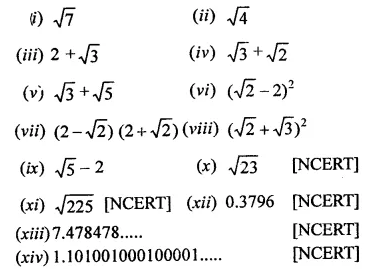Solution: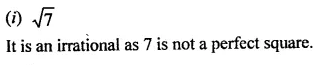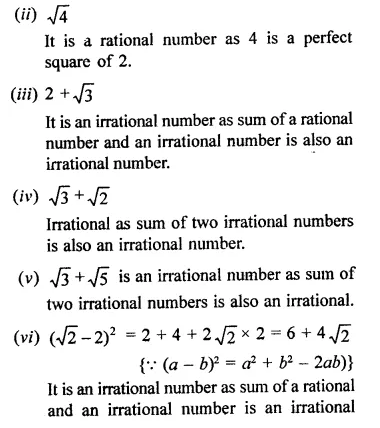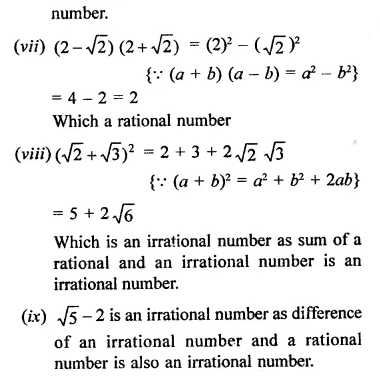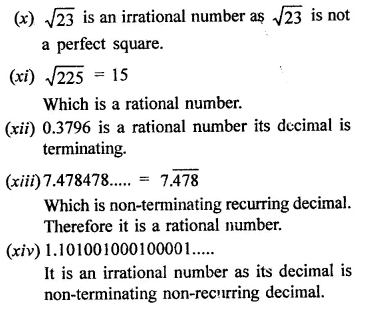Question 4.
Identify the following as rational or irrational numbers. Give the decimal representation of rational numbers: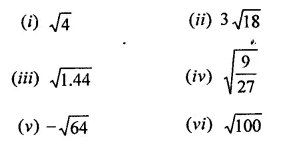Solution: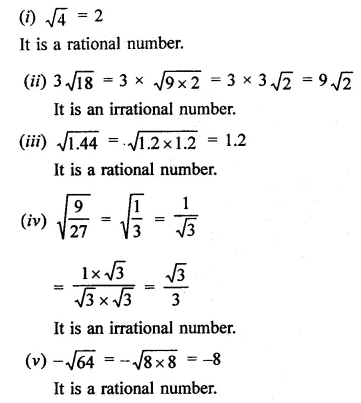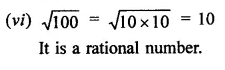Question 5.
In  the following equation, find which variables x, y, z etc. represent rational or irrational numbers: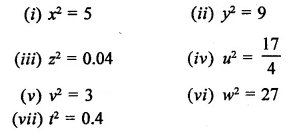Solution: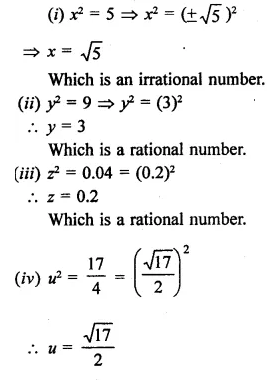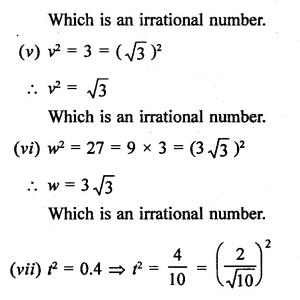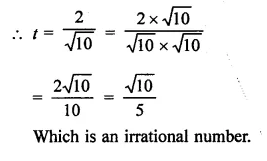Question 6.
Given two rational numbers lying between 0.232332333233332… and 0.212112111211112.
Solution:
Two rational numbers lying between 0.232332333233332… and 0.212112111211112… will be 0.232 and 0.212

Question 7.
Give two rational numbers lying between 0.515115111511115… and 0.5353353335…
Solution:
Two rational numbers lying between 0.515115111511115… and 0.535335333533335… will be 0.515, 0.535

Question 8.
Find one irrational numbers between 0.2101 and 0.2222… = 0.$$\overline { 2 }$$.
Solution:
One irrational number lying between 0.2101 and 0.2222… = 0.$$\overline { 2 }$$ will be 2201.0010001…

Question 9.
Find a rational number and also an irrational number lying between the numbers, 0.3030030003… and 0.3010010001…
Solution:
Between two numbers 0.3030030003… and 0.3010010001…, a rational will be 0.301 and irrational number will be 0.3020020002…

Question 10.
Find three different irrational numbers between the rational numbers $$\frac { 5 }{ 7 }$$ and $$\frac { 9 }{ 11 }$$. [NCERT]
Solution: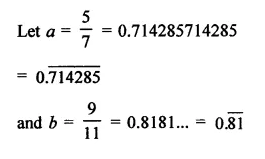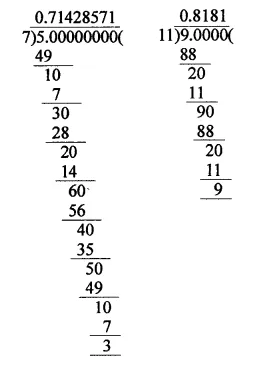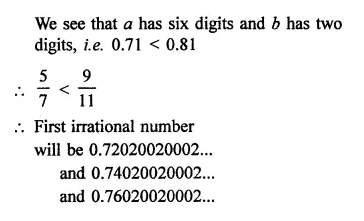Question 11.
Give an example of each, of two irrational numbers whose:
(i) difference is a rational number.
(ii) difference is an irrational number.
(iii) sum is a rational number.
(iv) sum is an irrational number.
(v) product is a rational number.
(vi) product is an irrational number.
(vii) quotient is a rational number.
(viii) quotient is an irrational number.
Solution:
(i) Two numbers whose difference is also a rational number, e.g. $$\sqrt { 2 }$$
, $$\sqrt { 2 }$$  which are irrational numbers.
∴ Difference = $$\sqrt { 2 }$$ – $$\sqrt { 2 }$$ = 0 which is also a rational number.
(ii) Two numbers whose difference is an irrational number.
e.g. $$\sqrt { 3 }$$  and $$\sqrt { 2 }$$  which are irrational numbers.
Now difference = $$\sqrt { 3 }$$  –$$\sqrt { 2 }$$  which is also an irrational number.
(iii) Let two irrational numbers be $$\sqrt { 3 }$$  and –$$\sqrt { 2 }$$  which are irrational numbers.
Now sum = $$\sqrt { 3 }$$  + (-$$\sqrt { 3 }$$) = $$\sqrt { 3 }$$
– $$\sqrt { 3 }$$  = 0 Which is a rational number.
(iv) Let two numbers be $$\sqrt { 5 }$$  , $$\sqrt { 3 }$$ which are irrational numbers.
Now sum = $$\sqrt { 5 }$$ + $$\sqrt { 3 }$$  which is an irrational number.
(v) Let numbers be $$\sqrt { 3 }$$  +$$\sqrt { 2 }$$and  $$\sqrt { 3 }$$  –$$\sqrt { 2 }$$which are irrational numbers.
Now product = ($$\sqrt { 3}$$  +$$\sqrt { 2 }$$ ) ($$\sqrt { 3 }$$ –$$\sqrt { 2 }$$)
= 3-2 = 1 which is a rational number.
(vi) Let numbers be $$\sqrt { 3 }$$ and $$\sqrt { 5 }$$ , which are irrational number.
Now product = $$\sqrt { 3 }$$ x $$\sqrt { 5 }$$  = $$\sqrt { 3×5 }$$
= $$\sqrt { 15 }$$
which is an irrational number.
(vii) Let numbers be 6 $$\sqrt { 2 }$$  and 2 $$\sqrt { 2 }$$ which are irrational numbers.
Quotient =$$\frac { 6\sqrt { 2 } }{ 2\sqrt { 2 } }$$ = 3 which is a rational number.
(viii) Let numbers be $$\sqrt { 3 }$$and $$\sqrt { 5 }$$ which are irrational numbers.
Now quotient =$$\frac { \sqrt { 3 } }{ \sqrt { 5 } }$$ = $$\sqrt { \frac { 3 }{ 5 } }$$ which is an  irrational number.

Question 12.
Find two irrational numbers between 0.5 and 0.55.
Solution:
Two irrational numbers between 0.5 and 0.55 will be 0.51010010001… and 52020020002…

Question 13.
Find two irrational numbers lying betwee 0.1 and 0.12.
Solution:
Two irrational numbers lying between 0.1 and 0.12 will be 0.1010010001… and 0.1020020002…

Question 14.
Prove that $$\sqrt { 3 }$$+$$\sqrt { 5 }$$ is an irrational number.
Solution: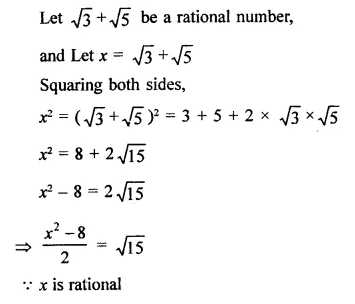Hope given RD Sharma Class 9 Solutions Chapter 1 Number Systems Ex 1.4 are helpful to complete your math homework.

If you have any doubts, please comment below. Learn Insta try to provide online math tutoring for you.